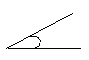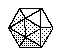Name: ___________________Date:___________________

 Email us to get an instant 20% discount on highly effective K-12 Math & English kwizNET Programs!

### Grade 3 - Mathematics8.19 Geometry Review Test

 Q 1: An angle that is less than a right angle is ______ angle.Answer: Q 2: Perimeter of an equilateral triangle =2 x side3 x side4 x side Q 3: Area of a rectangle = 2 x area of a triangle =TrueFalse Q 4: If you put two points on a paper and connect them you will have alineraysegment Q 5: A 3D figure with no vertices or sidesconespherecylinder Q 6: A line that goes completely through a circle is adiameterrayradius Q 7: What is the shape with 20 faces, each formed by an equilateral triangle?icosahedrontwentyhedronhydron Q 8: What is an angle that forms a square corner.abtuserightacute Question 9: This question is available to subscribers only! Question 10: This question is available to subscribers only!